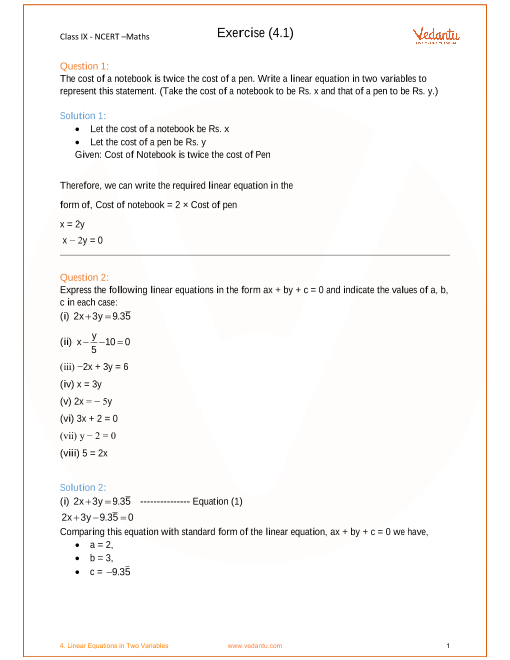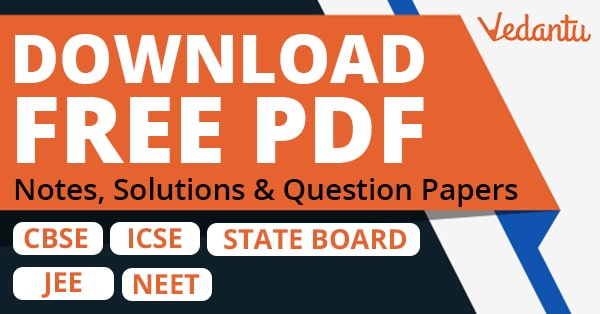# NCERT Solutions for Class 9 Maths Chapter 4 Linear Equations in Two Variables (Ex 4.4) Exercise 4.4

## NCERT Solutions for Class 9 Maths Chapter 4 Linear Equations in Two Variables (Ex 4.4) Exercise 4.4

Free PDF download of NCERT Solutions for Class 9 Maths Chapter 4 Exercise 4.4 (Ex 4.4) and all chapter exercises at one place prepared by expert teacher as per NCERT (CBSE) books guidelines. Class 9 Maths Chapter 4 Linear Equations in Two Variables Exercise 4.4 Questions with Solutions to help you to revise complete Syllabus and Score More marks. Register and get all exercise solutions in your emails.